Concentric circles

There is given a circle K with a radius r = 8 cm. How large must a radius have a smaller concentric circle that divides the circle K into two parts with the same area?

Result

r2 =  5.657 cm

Solution:Leave us a comment of example and its solution (i.e. if it is still somewhat unclear...):Be the first to comment!Next similar examples:

1. Circular lawnAround a circular lawn area is 2 m wide sidewalk. The outer edge of the sidewalk is curb whose width is 2 m. Curbstone and the inner side of the sidewalk together form a concentric circles. Calculate the area of the circular lawn and the result round to 1
2. Holidays - on poolChildren's tickets to the swimming pool stands x € for an adult is € 2 more expensive. There was m children in the swimming pool and adults three times less. How many euros make treasurer for pool entry?
3. An equilateral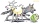An equilateral triangle with a side 10 m represents a wooden platform standing in a lawn. A goat is tied to a corner with a 15 m rope. What is the maximum amount of grazing area available to the goat?
4. Regular octagonDraw the regular octagon ABCDEFGH inscribed with the circle k (S; r = 2.5 cm). Select point S' so that |SS'| = 4.5 cm. Draw S (S '): ABCDEFGH - A'B'C'D'E'F'G'H'.
5. Wiring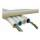Conduit has a cross section 482 mm. Maybe put it into 6 conductors with a cross section S2 \$mm2?
6. Silver medal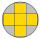To circular silver medal with a diameter of 10 cm is inscribed gold cross, which consists of five equal squares. What is the content area of silver part?
7. Inscribed circle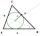The circle inscribed in a triangle has a radius 3 cm. Express the area of the triangle using a, b, c.
8. AceThe length of segment AB is 24 cm and the point M and N divided it into thirds. Calculate the circumference and area of this shape.
9. DiameterWhat is the inside diameter of the cylinder container and if half a liter of water reaches a height 15 cm?
10. Pipe cross sectionThe pipe has an outside diameter 1100 mm and the pipe wall is 100 mm thick. Calculate the cross section of this pipe.
11. Area to perimeterCalculate circle circumference if its area is 254.34cm2
12. Disc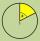Circumference of the disk is 78.5 cm. What is the circumference of the circular arc of 32° on the disc?
13. Roof tilesThe roof has a trapezoidal shape with bases of 15 m and 10 m, height of roof is 4 meters. How many tiles will need if on 1 m2 should be used 8 tiles?
14. TrapeziumThe area of trapezium is 35 cm2. Find its altitude if the bases are 6cm and 8 cm.
15. Land areas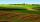Two land areas is 244 m2. The first parcel is 40 m2 less than twice of the second one. What have acreage of each parcel?
16. Rhombus diagonalArea of rhombus is 224. One diagonal measures 33, find length of other diagonal.
17. TrapeziumThe area of trapezium is 35cm2 find its altitude if the bases are 6cm and 8cm.